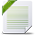Decision Optimization

View Only

How to get access to the coefficient matrix in a QCQP model that already been built in the C++ API?Musheng Li16 days agoVincent Beraudier12 days agoMusheng Li10 days agoVincent Beraudier9 days ago• 1.  How to get access to the coefficient matrix in a QCQP model that already been built in the C++ API?

Posted 16 days ago
Hi Everyone,

I am solving a Quadratic Programming Problem with Quadratic Constraints (QCQP) with CPLEX using C++ API. Currently, I am struggling with getting access to the coefficient matrix in a model that has already been built. The objective function of the model is quadratic, and the constraints include Second-order cone programming (SOCP) constraints, Quadratic constraints, and linear constraints.
And I have the following ideas:
1) Use IloModel::Iterator to traverse the variables, constraints, and objectives in the model.
2) Use IloExtractable to judge their types.
I wonder if this idea is correct, and Can anyone provide a piece of C + + code for reference?

------------------------------
Musheng Li
------------------------------

• 2.  RE: How to get access to the coefficient matrix in a QCQP model that already been built in the C++ API?

Posted 12 days ago
Hello, I think it would be something like
while (it.ok()) {
IloNum c = it.getCoef();
IloNumVar v1 = it.getVar1();
IloNumVar v2 = it.getVar2();
std::cout << c <<  "*" << v1 << "*" << v2 << "\n";
++it;
}
}
void iterate(IloModel model){
for (IloModel::Iterator it(model); it.ok(); ++it) {
IloExtractable ext = *it;
if (ext.isObjective()) {
IloObjective o = ext.asObjective();
std::cout << "  ===== start obj ====\n";
iterate(it);
std::cout << "  ===== end obj ====\n";
}
else if (ext.isConstraint()) {
IloConstraint c = ext.asConstraint();
if (c.getImpl()->isType(IloRangeI::GetTypeInfo())){
IloRange r = (IloRangeI*)c.getImpl();
std::cout << "  ===== start range ====\n";
iterate(it);
std::cout << "  ===== end range ====\n";
}
}
}
}
​

------------------------------
Vincent Beraudier
------------------------------

• 3.  RE: How to get access to the coefficient matrix in a QCQP model that already been built in the C++ API?

Posted 10 days ago
Thanks a lot for your reply, your code is inspiring to me, and I've figured it out.
But now I have another problem. When I try to read the constant term in the objective function of a MIQP problem, I.e. miqpex1.lp, the getConstant() function fails to read the value. In order to prove the correctness of the code, I use it to read the constant term of a SOCP model, that is ilosocpex1.lp, the program outputs the correct value 0. I checked a lot of official documents, but still can't figure out why.

See the program in obj_test.cpp
Please refer to miqpex1.lp for the MIQP model
See ilosocpex1.lp for the SOCP model

A Example of the function call:
obj_test miqpex1.lp

------------------------------
Musheng Li
------------------------------

Attachment(s)obj_test.cpp   797 B 1 versionmiqpex1.lp   321 B 1 versionilosocpex1.lp   283 B 1 version

• 4.  RE: How to get access to the coefficient matrix in a QCQP model that already been built in the C++ API?

Posted 9 days ago
It's a limitation (or a bug) in concert, which checks if the objective is complex in term of expressions or not (imbricated expressions can be hard to normalize), so an exception is raised.
We will look to improve this check in a next version.
Meanwhile, a code which should be safe is as follows:
if (e.isObjective()) {
IloObjective obj = e.asObjective();
IloObjectiveI* o = obj.getImpl();
IloNumLinTermI* e = o->getExpr();
if (e->getQuad() && e->getFirstGeneral() == e->getLastGeneral()) {
std::cout << "Obj " << e->getNumConstant() << "\n";
}
else {
std::cout << "standard obj\n";
std::cout << "Obj " << obj.getConstant() << "\n";
}
}​

------------------------------
Vincent Beraudier
------------------------------

• 5.  RE: How to get access to the coefficient matrix in a QCQP model that already been built in the C++ API?

Posted 9 days ago
Thanks for your prompt reply, the code that you provide works well for me, thanks again！

------------------------------
Musheng Li
------------------------------